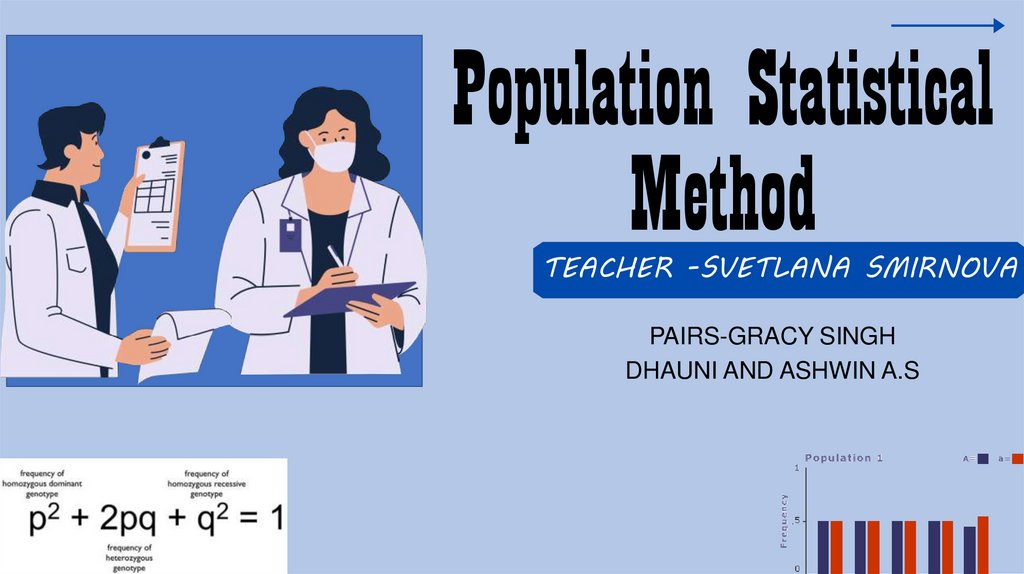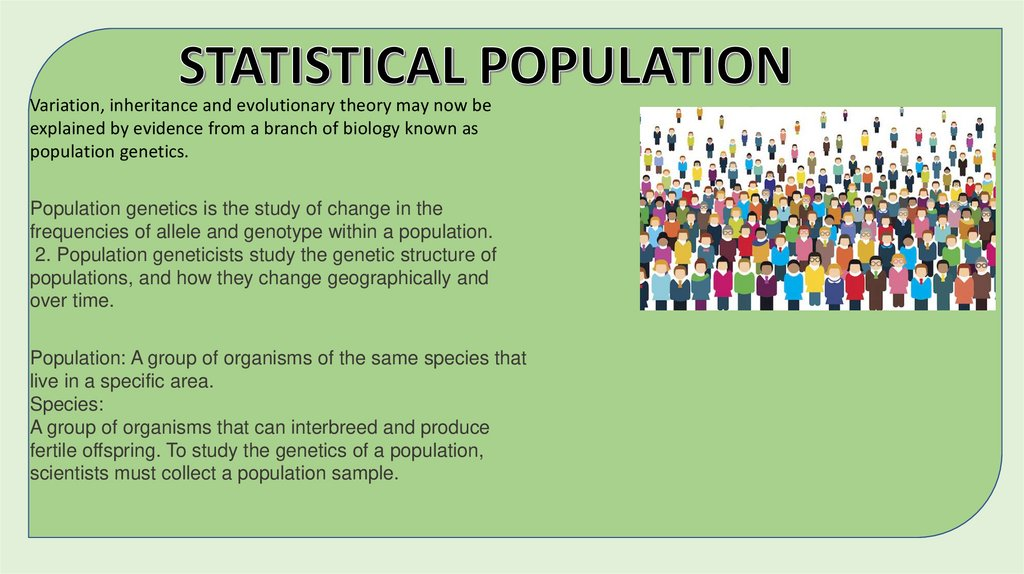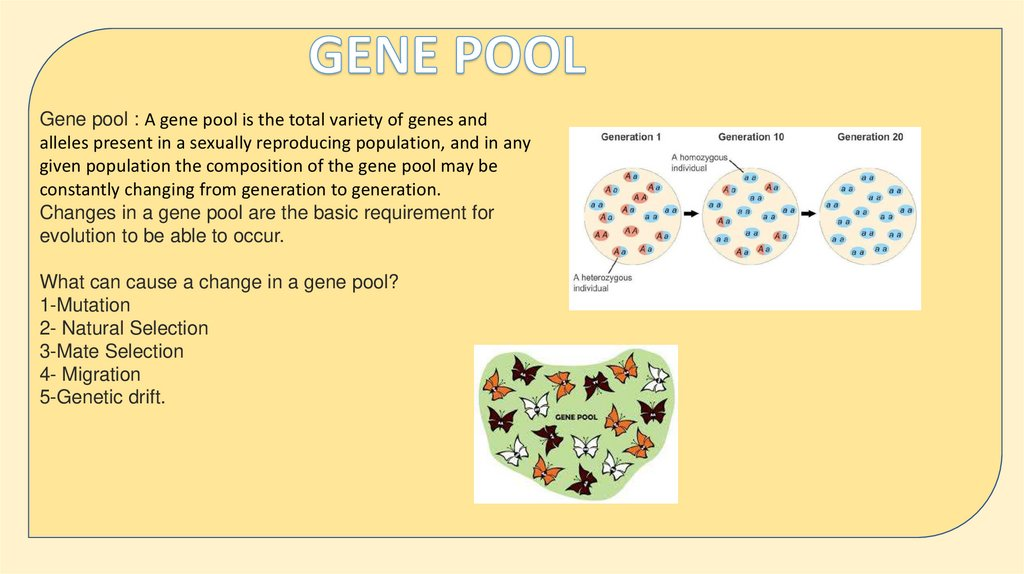# Population statistics

## 1.

Population Statistical
Method
TEACHER -SVETLANA SMIRNOVA
PAIRS-GRACY SINGH
DHAUNI AND ASHWIN A.S

## 2.

Variation, inheritance and evolutionary theory may now be
explained by evidence from a branch of biology known as
population genetics.
Population genetics is the study of change in the
frequencies of allele and genotype within a population.
2. Population geneticists study the genetic structure of
populations, and how they change geographically and
over time.
Population: A group of organisms of the same species that
live in a specific area.
Species:
A group of organisms that can interbreed and produce
fertile offspring. To study the genetics of a population,
scientists must collect a population sample.

## 3.

Gene pool : A gene pool is the total variety of genes and
alleles present in a sexually reproducing population, and in any
given population the composition of the gene pool may be
constantly changing from generation to generation.
Changes in a gene pool are the basic requirement for
evolution to be able to occur.
What can cause a change in a gene pool?
1-Mutation
2- Natural Selection
3-Mate Selection
4- Migration
5-Genetic drift.

## 4.

Allele frequency- The appearance of any physical characteristic,
for example coat color in mice, is determined by one or more
genes. Several forms of each gene may exist and these are
called alleles. The number of organisms in a population
carrying a particular allele determines the allele frequency
(which is sometimes, incorrectly, referred to as the gene
frequency).

## 5.

Genotype frequencies –
The frequencies of particular alleles in the gene pool are of
importance in calculating genetic changes in the population
and in determining the frequency of genotypes. Since the
genotype of an organism is the major factor determining its
phenotype, calculations of genotype frequency are used in
predicting possible outcomes of particular matings or crosses.
This has great significance in horticulture, agriculture and
medicine.

## 6.

• Scientists monitor frequency of the dominant and recessive allele in a
population year after year.
• In other words, scientists may monitor the values of p and q for a
population over several generations.
• If the values of p and q… -remain the same the population is not evolving
according to scientists. -change then scientist say the group is evolving.
• Evolution- “genetic change” amongst population
• Scientist argue all populations will evolve unless “certain conditions” are
upheld within the group

## 7.

The mathematical relationship between the frequencies of alleles and
genotypes in populations was developed independently in 1908 by an English
mathematician G. H. Hardy and a German physician W. Weinberg.
The relationship known as the Hardy-Weinberg equilibrium is based upon a
principle, which states that «the frequency of dominant and recessive alleles in
a population will remain constant from generation to generation provided
certain conditions exist»
These conditions are:
(1) the population is large;
(2) mating is random;
(3) no mutations occur;
(4) all genotypes are equally fertile, so that no selection occurs;
(5) generations do not overlap;
(6) there is no emigration or immigration from or into the population, that is,
there is no gene flow between populations.

## 8.

HARDY WINBERG CONDITIONS1. No mutations
2. No individual can be more adapted to survive than
any other (no “survival fittest”).
3. The population must be large and stay the same size
(no immigration or emigration).
4. Mating must be completely random.

## 9.

• How do scientists determine values of p and q in a
population?
• First, they collect a population sample.
• For example, 100 racerunners of which 85 have
white stripes and 15 yellow stripes.
• What is the genotype 85 with white stripes? -TT or Tt
• What is the genotype of 15 with yellow stripes? -tt
Using this information, scientists can find values for
p and q of the population.

## 10.

In terms of genotype frequency the sum of the three genotypes presented in the
population equal one, or, expressed in terms of the symbols p and q, it can be seen
that the genotypic probabilities are:
p 2 + 2pq + q 1 = 1
(In mathematical terms p + q = 1 is the mathematical equation of probability and p 2
+ 2pq + q 2 = 1 is the binomial expansion of that equation (that is (p + q)2 ).
To summarise, since
p = dominant allele frequency
q = recessive allele frequency
p 2 = homozygous dominant genotype
2pq = heterozygous genotype
q 2 = homozygous recessive genotype
it is possible to calculate all allele and genotype frequencies using the expressions:
allele frequency
p + q =1,
and genotype frequency
p 2 + 2pq + q 2 = 1.

## 11.

The Hardy-Weinberg equation Whilst
the Hardy-Weinberg equation provides
a simple mathematical model of how
genetic equilibrium can be maintained
in a gene pool, its major application in
population genetics is in calculating
allele and genotype frequencies.

## 13.

• The Hardy-Weinberg model enables us
to compare a population's actual genetic
structure over time.
• If genotype frequencies differ from
those we would expect under equilibrium,
we can assume that one or more of the
model's assumptions are being violated.

## 14.

• The correctness of the frequencies can be verified by
the substitution of the values in the equations p + q=1 p2
+ 2pq + q2 = 1
• Thus genotype frequencies at the EST locus are in
Hardy-Weinberg equilibrium.
• We can expect these allele frequencies to remain
constant over time.
• This equilibrium in the genetic structure of the
population at the EST locus indicates that this particular
locus is not changing. Hence the population is not
evolving.

## 15.

• One or more of the assumptions are violated in most
situations.
• Most populations are under the influence of natural
selection.
• Many populations are not even large enough to be
functionally infinite.
• Oftentimes populations are not completely isolated
from one another and migration of individuals into or out
of one population can change its genetic makeup.

## 16.

WORKSHEETKeerthana -What is Gene pool ?
Explain Hardy-Weinberg equilibrium
Nidhi -Factors affecting hardy Weinberg equilibrium
What you mean by Hardy-Weinberg equation
Sakhi -Importance of frequencies of particular alleles in the gene pool
Explain Allele?
Ekta - What is allele frequency ?
How does Allele frequency affect gene pool ?
Teena- Explain population genetics?
What is the main purpose of population genetics?
Karmshil - Significance of Hardy - Weinberg equation ?
explain hardy Weinberg principle?
Amit – what are the hardy Weinberg conditions?
what do you understand by genetic frequencies?
Aishwary – Write the hardy Weinberg equations?
How do scientists determine p and q?
Hari Shankar – write the limitations of Hardy Weinberg principle?
How do scientist monitor the frequency?
Vikram – Explain population?
what do you understand by population genetics?
Harish – What do you understand by gene pool?
what is genotypic frequency?